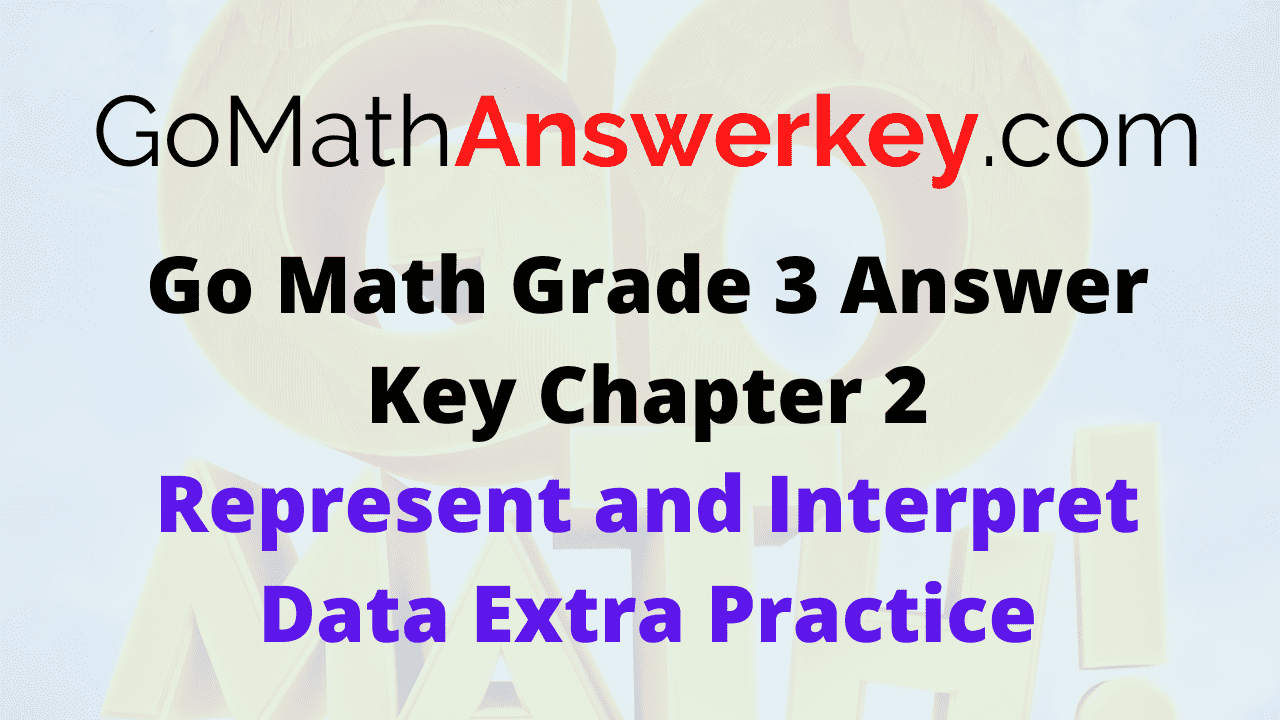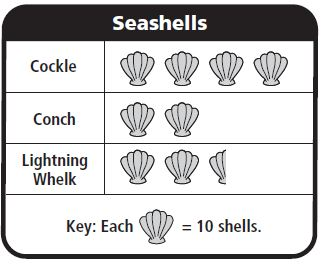# Go Math Grade 3 Answer Key Chapter 2 Represent and Interpret Data Extra Practice

Go Math Grade 3 Answer Key Chapter 2 Represent and Interpret Data Extra Practice helps the students to secure the highest marks in the exam. Learn the simple tricks to solve the problems from Go Math Grade 3 Answer Key. Refer the solution given in the Go Math Grade 3 Chapter 2 Answer Key Extra Practice to know whether the answer is correct or not.

## Go Math Grade 3 Answer Key Chapter 2 Represent and Interpret Data Extra Practice

Before solving the problems first learn the methods. There are several methods to solve the problems in maths. So, check out the methods provided in the Go Math Solution Key Chapter 2 Represent and Interpret Data Extra Practice for better understanding.

### Common Core – Page No. 45000

Lesson 2.1

Use the Pets tables for 1–2.

Question 1.
Manny collected data about pets owned by students in his class. Complete Manny’s tally table and frequency table.

 Pets Pets Tally Cat ________ Dog ________ Bird ________ Fish ________

 Pets Pets Tally Cat |||| Dog || Bird | Fish |

Question 1.

 Pets Pets Frequency ______ 4 ______ 2 ______ 1 ______ 1

 Pets Pets Frequency Cat 4 Dog 2 Bird 1 Fish 1

Question 2.
How many more students have cats than have dogs and birds combined?
______ students

Explanation:

Number of students who have cats = 4
Number of students who have Dogs = 2
Number of students who have Birds = 1
Total students who have Dogs and birds combined = 2 + 1 = 3
To know how many more students have cats than have dogs and birds combined, we need to subtract students who have Dogs and birds combined from the Number of students who have cats.
4 – 3 = 1

Lessons 2.2–2.3

Use the Seashells picture graph for 3–5.Question 3.
Maggie has a picture graph that shows the seashells she collected. How many seashells did Maggie collect in all?
______ seashells

Explanation:

Number of Cockle shells =4Each= 10 shells
So, Number of Cockle shells = 10 + 10 + 10 + 10 = 40 shells
Number of Conch Shells = 2Each= 10 shells
= 10 + 10 = 20 shells
Number of Lightning Whelk = 2 and a halfEach= 10 shells
= 10 + 10 + 5 = 25 shells

Total number of shells that Maggie collected = 40 + 20 + 25 = 85 shells

Question 4.
How many more cockle shells did she collect than lightning whelks?
______ shells.

Explanation:

Number of Cockle shells =4Each= 10 shells
So, Number of Cockle shells = 10 + 10 + 10 + 10 = 40 shells
Number of Lightning Whelk = 2 and a half= 10 + 10 + 5 = 25 shells
Subtract Number of Lightning Whelk shells from Number of Cockle shells
= 40 – 25 = 15 shells

Question 5.
What if the key were “Each= 5 shells?” How many pictures would there be for conch?
______ pictures

Explanation:

Number of Conch Shells = 2If each= 5 shells
Then the number of Conch shells will be 4= 5 + 5 + 5 + 5 = 20 shells

Page No. 46000

### Common Core

Lessons 2.4–2.6

Use the Bicycle Rides frequency table for 1–3.Question 1.
The frequency table shows the number of miles Sean rode on his bicycle. Use the data in the frequency table to complete the bar graph.
Type below:
_________

Question 2.
How many more miles did Sean ride on Saturday than on Monday?
______ miles

Explanation:

Number of miles that Sean ride on Saturday = 12
Number of miles that Sean ride on Monday = 4
To find how many more miles did Sean ride on Saturday than on Monday
Subtract the number of miles that Sean ride on Monday from Number of miles that Sean ride on Saturday
12 – 4 = 8 miles

Question 3.
Write a number sentence to show how many miles in all Sean rode on his bicycle.
______ miles

Explanation:

Number of miles that Sean ride on Saturday = 12
Number of miles that Sean ride on Monday = 4
Number of miles that Sean ride on Wednesday = 9
Total number of miles that Sean ride = 12 + 9 + 4 = 25 miles

Lesson 2.7

Use the Number of Beads line plot for 4–6.Question 4.
Kim is making bead necklaces. She records the number of beads on the different necklaces on a line plot. How many necklaces have exactly 50 beads?
______ necklaces

Explanation:

The line plot shows that there are 5 necklaces that have exactly 50 beads.

Question 5.
How many necklaces have fewer than 40 beads?
______ necklaces

Explanation:

The above figure shows that the number of necklaces that have less than 40 beads = 3

Question 6.
How many necklaces have 50 or more beads?
______ necklaces